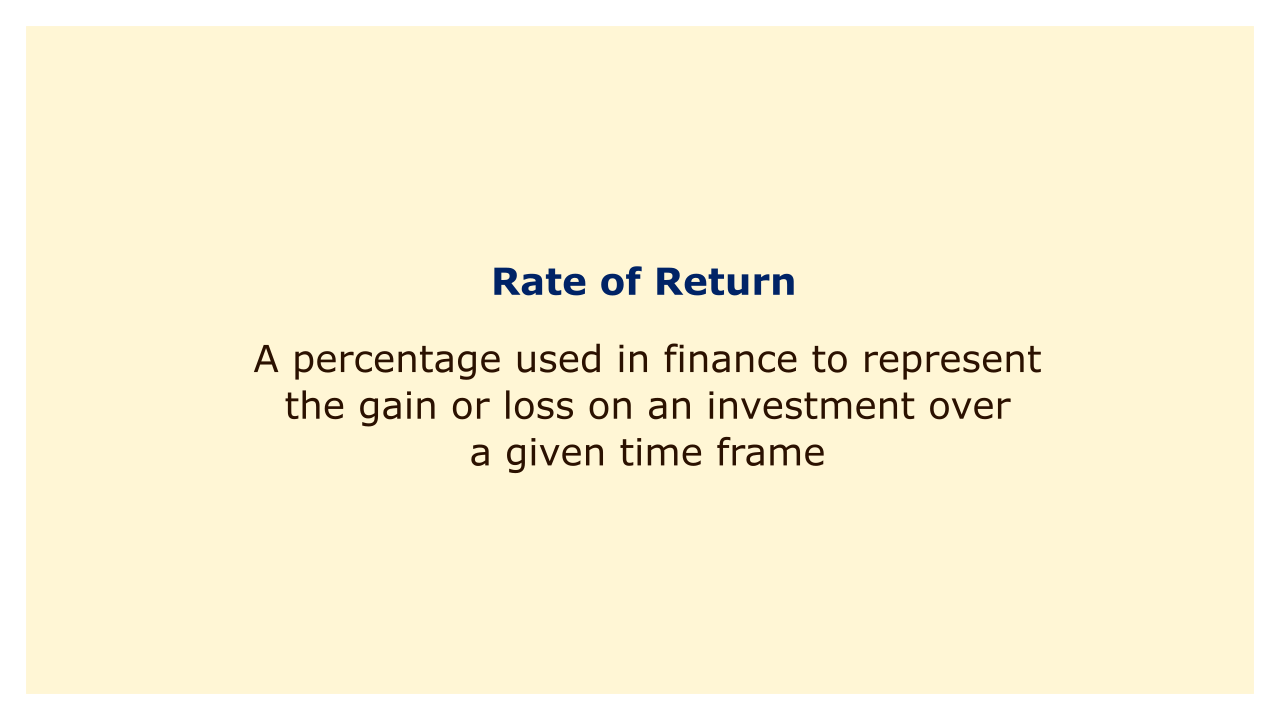# Rate of ReturnImage: Moneybestpal.com

### The rate of return is a percentage used in finance to represent the gain or loss on an investment over a given time frame. It is frequently used to assess investment performance and to contrast various investment opportunities.

Depending on the type of investment and return being measured, different formulas can be used to calculate the rate of return.

The simple return formula, which assesses the percentage change in an investment's value from the start to the finish of a specified period, is one popular method for calculating the rate of return. The simple return for the year is 10%, for instance, if an investment starts out at \$10,000 and ends up being worth \$11,000.

The compound return formula, which accounts for compounding's impact, is another method for calculating the rate of return. The rate of return is calculated using this formula under the assumption that all profits are reinvested in the original investment. When analyzing long-term investments like stocks or mutual funds, where returns are frequently reinvested, the compound return formula is typically used.

Real or nominal numbers can be used to express the rate of return. The nominal return on an investment represents the actual return received, whereas the real return takes inflation into account and reflects the investment's buying power.
Tags